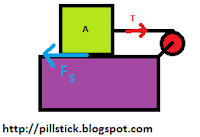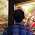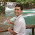• Instagram

Mechanical #Problem : 1

Hello guys, this time I have a mechanical problem. See this picture

Given that :
• The purple block is not move.
• $M_{A} < M_{B}$
• Gravitational acceleration = $g$
• The kinetic frictional cooficient = $\mu _k$

1. If the block move to down, determine the acceleration and the string tension.

Hold on guys !!!
Stop on this line !!!
Do it by yourself before you look the key answer and the solution

Number 1

$a = \frac {(m_{B} - m_{A} . \mu_{k})}{(m_{A} + m_{B})}g$

$T = \frac {m_{A} . m_{B} . (\mu_{k} + 1)} {(m_{A} + m_{B})}g$

Explanation:
First of all, we can divided that system into two dependent systems. See picture belowSystem 1

From system 1, we got :
$\begin{eqnarray*} \Sigma F &=& m_{A} . a \\ T - F_{k} &=& m_{A} . a \\ T - W_{A} . \mu _{k} &=& m_{A} . a \\ T - m_{A} . g . \mu _{k} &=& m_{A} . a \cdots (1) \end{eqnarray*}$System 2

From system 2, we got :
$\begin{eqnarray*} \Sigma F &=& m_{B} . a \\ W_{B} - T &=& m_{B} . a \\ m_{B} . g - T &=& m_{B} . a \cdots (2) \end{eqnarray*}$

Then, eliminate $(1)$ and $(2)$.
If we add them, we will get :
$\begin{eqnarray*} T - m_{A} . g . \mu _{s} &=& m_{A} . a \\ m_{B} . g - T &=& m_{B} . a \\ m_{B} . g - m_{A} . g. \mu_{k} &=& (m_{A} + m_{B}) . a \\ a &=& \frac {(m_{B} - m_{A} . \mu_{k})}{(m_{A} + m_{B})}g\end{eqnarray*}$

Then, we can determine the tension. See it : ...
$\begin{eqnarray*}T - m_{A} . g . \mu _{k} &=& m_{A} . a \\ T &=& m_{A} . g . \mu_{k} + m_{A} . \frac {(m_{B} - m_{A} . \mu_{k})}{(m_{A} + m_{B})}g \\ T &=& m_{A} . g . \frac {\mu_{k}.(m_{A} + m_{B}) + (m_{B} - m_{A} . \mu_{k})} {(m_{A} + m_{B})}\\ T &=& m_{A} . g . \frac {\mu_{k}.m_{A} + \mu_{k}.m_{B} + m_{B} - m_{A} . \mu_{k}} {(m_{A} + m_{B})}\\ T &=& m_{A} . g . \frac {\mu_{k}.m_{B} + m_{B}} {(m_{A} + m_{B})}\\ T &=& \frac {m_{A} . m_{B} . (\mu_{k} + 1)} {(m_{A} + m_{B})}g\\ \end{eqnarray*}$

1.Mantap, tambah soal lebih banyak lagi . . .

1.2.Hey Cahya, can you make a post about mirror and lens? i hope you can, because i will have a test soon. But if you can't, no problem... :)
Visit back me okay

1.sorry, i'm afraid i can't make it right on time, i suggest you go to this link.

Hey, It's my pleasure to know what was in your mind after reading the article above. So, you can comment or give critics to my writing on this comment box below